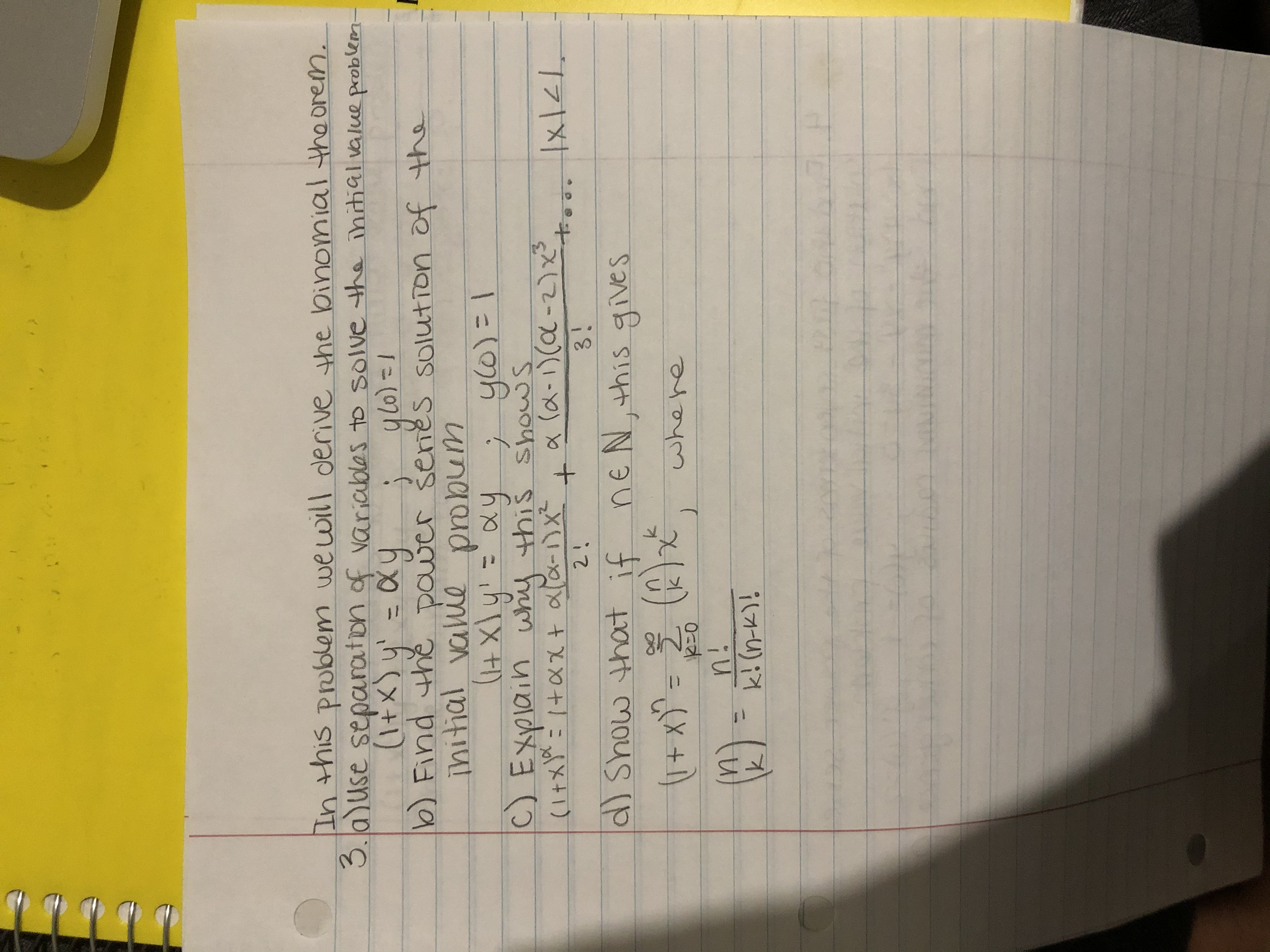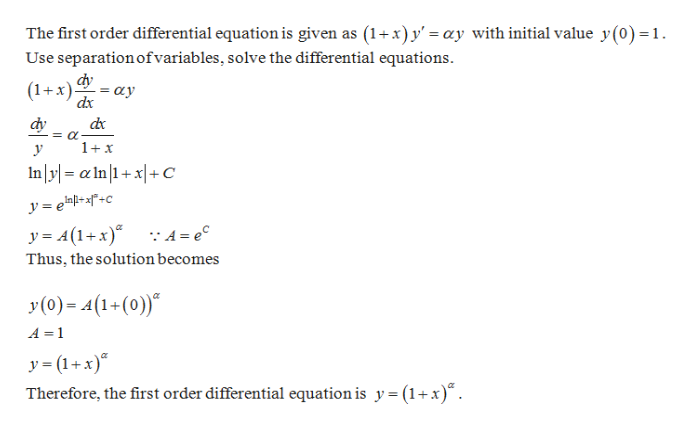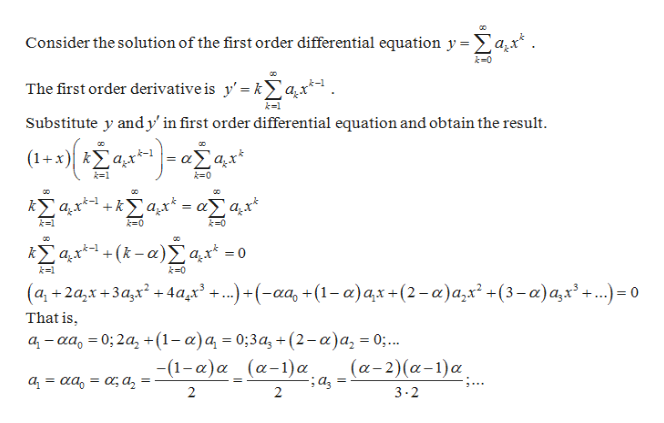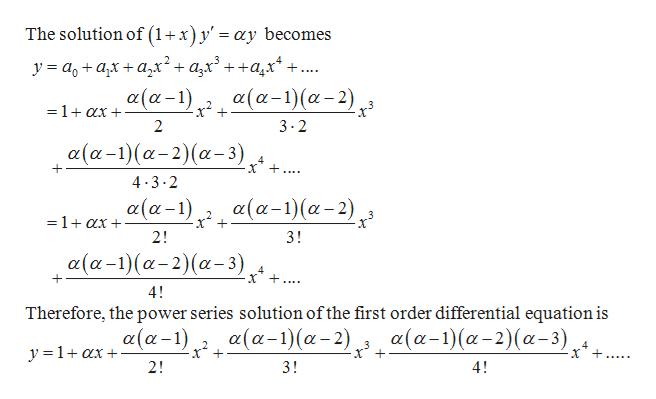# In this poblem wewill derive the loinomial thaorem.3. a)use separaton of variablas to solve the ihrtial value probleen-(4X)y-ay0) Find the paver senes solution of theinitial vaie probume l11(tXIy yC) Explain why this shows(Itx +axt ala-x (a-1(a-2)+171x13!2 1d) Show that ifne Nthis giveswhere20n!k! (n-K)

Question
24 views

3help_outlineImage TranscriptioncloseIn this poblem wewill derive the loinomial thaorem. 3. a)use separaton of variablas to solve the ihrtial value probleen- (4X)y-ay 0) Find the paver senes solution of the initial vaie probum e l 11 (tXIy y C) Explain why this shows (Itx +axt ala-x (a-1(a-2) + 171x1 3! 2 1 d) Show that if ne Nthis gives where 20 n! k! (n-K) fullscreen
check_circle

Step 1

3 a]help_outlineImage TranscriptioncloseThe first order differential equation is given as (1+x) y' = ay with initial value y(0) =1 Use separation ofvariables, solve the differential equations dy (1+x) ay dx dy dc 1+x In yl= aIn|1+x|+C y= ep+zf°+c_ y= 4(1+x) :A= ec Thus, the solution becomes y(0)4(1-(0) A = 1 y =(1+x)® Therefore, the first order differential equation is y = (1+x) fullscreen
Step 2

3 b]help_outlineImage TranscriptioncloseConsider the solution of the first order differential equation y =a^x* . k-0 The first order derivative is y' = kax' k=1 Substitute y andy' in first order differential equation and obtain the result (1+ x ka^x* k1 + = k-0 Σαr-(& - α)Σ α' - + kl k-0 (a, 2a,x+3a,x+ 4ax +..) +(-aa, +(1- a)ax+(2-a)a,x +(3-a)a,x'...)= o That is a-aa 0; 2a +(1-a)a = 0;3a, + (2 - a)a, = 0;.. -(1-a)a_(a-1) a (α-2)(α-1)α, α- αα,-αα aa a2 2 2 3-2 fullscreen
Step 3

Thus,

...help_outlineImage TranscriptioncloseThe solution of (1x)y' = ay becomes y = ao+a,x + azx2+a,x'++a.x*- +... α(α-1)- α(α-1) (α-2), =1+ax 2 3-2 α(α-1) (α-2) (α-3) , .. 4 3.2 α(α-1),, α(α-1)(α-2), =1+ax 2! 3! α (α-1) (α- 2)(α- 3) ... 4! Therefore, the power series solution of the first order differential equation is α(α-1) (α-2) α (α-1) α (α-1) (α-2) (α-3) y 1ax 2! 3! 4! fullscreen

### Want to see the full answer?

See Solution

#### Want to see this answer and more?

Solutions are written by subject experts who are available 24/7. Questions are typically answered within 1 hour.*

See Solution
*Response times may vary by subject and question.
Tagged in

### Math